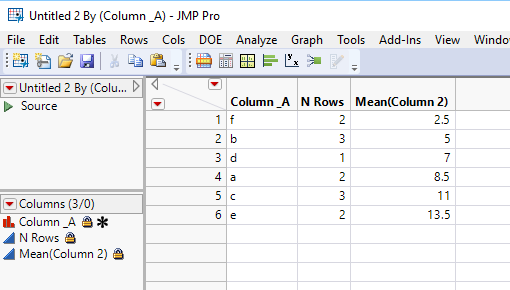Our World Statistics Day conversations have been a great reminder of how much statistics can inform our lives. Do you have an example of how statistics has made a difference in your life? Share your story with the Community!
Choose Language Hide Translation Bar
Highlighted

## Summarize Without Alphabetizing

I have a dataset where the order is important and I want to be able to summarize a character column with repeat values without the results being in alphabetical order.

column_A

f

f

b

b

b

d

a

a

c

c

c

e

e

Usual methods return {a,b,c,d,e,f},

I am trying to get {f,b,d,a,c,e}

2 ACCEPTED SOLUTIONS

Accepted Solutions
Highlighted

## Re: Summarize Without Alphabetizing

Sometimes, just by asking you end up freeing your mind to possible solutions. I give to you, the non-alphabetizing summarizer:

``````no_sort_summarize = function({list},
design_matrix = Design( list, list ); //compares the list to itself returning a 1 in the position of the first match for each repetition
summarized_matrix = (Transpose(V Sum(design_matrix))); //flatten matrix into one row and transpose into columns
unique_value_positions = Loc(summarized_matrix); //return only the position of the first instance of a value thus creating a summary
return(list[unique_value_positions]); //subscript list to return the non-sorted summarized values
);``````

Highlighted

## Re: Summarize Without Alphabetizing

I can officially confirm that Summarize() does now support the Value Ordering property as of JMP 14 and later.

8 REPLIES 8
Highlighted

## Re: Summarize Without Alphabetizing

Sometimes, just by asking you end up freeing your mind to possible solutions. I give to you, the non-alphabetizing summarizer:

``````no_sort_summarize = function({list},
design_matrix = Design( list, list ); //compares the list to itself returning a 1 in the position of the first match for each repetition
summarized_matrix = (Transpose(V Sum(design_matrix))); //flatten matrix into one row and transpose into columns
unique_value_positions = Loc(summarized_matrix); //return only the position of the first instance of a value thus creating a summary
return(list[unique_value_positions]); //subscript list to return the non-sorted summarized values
);``````

Highlighted

## Re: Summarize Without Alphabetizing

If the order you want to summarize by is the order found in the data, you can set the column property, "Row Order Level" for Column_A, and then run

Tables==>Summary

and the data will be summarized in the order the grouping column was found in the data tableJim
Highlighted

## Re: Summarize Without Alphabetizing

And, more generally, you can use the Value Ordering column property to impose whatever order on the variable you wish.  That order will be used in any subsequent analysis (tablulation, graphs, etc.).

HighlightedJeff_PerkinsonCommunity Manager

## Re: Summarize Without Alphabetizing

Unfortunately, in JMP 13 and earlier the Summarize() JSL function doesn't respect the Value Ordering property.

I've confirmed that this will be corrected in JMP 14.

-Jeff
Highlighted

## Re: Summarize Without Alphabetizing

Another reason I'm glad I rarely script anything - Tabulate works just fine with Value Ordering.

Highlighted

## Re: Summarize Without Alphabetizing

I can officially confirm that Summarize() does now support the Value Ordering property as of JMP 14 and later.

Highlighted

## Re: Summarize Without Alphabetizing

Hi Jeff,

I have JMP 14, but my summarize() function is still alphabetic, does the non-alphabetic version have another name?

Philippe

Highlighted

## Re: Summarize Without Alphabetizing

I have tried setting the Value Ordering column property for both numeric and character columns under JMP 14.3 and the Summarize() function uses the Value Ordering property as it's order.

Jim
Article Labels

There are no labels assigned to this post.